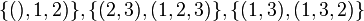# S2 is not normal in S3

This article gives the statement, and possibly proof, of a particular subgroup or type of subgroup (namely, Cyclic group:Z2 (?)) not satisfying a particular subgroup property in a particular group or type of group (namely, Symmetric group:S3 (?)).

## Statement

Let$G$ be the symmetric group:S3, which is the group of all permutations on$\{ 1,2,3 \}$. The group has the following six elements, given by their cycle decompositions:$\! \{ (), (1,2), (2,3), (1,3), (1,2,3), (1,3,2) \}$

Let$H$ be the subgroup$\{ (), (1,2) \}$. In other words,$H$ is the symmetric group of degree two embedded inside$G$.

Then,$H$ is not a normal subgroup of$G$.

To explore more, see element structure of symmetric group:S3 and subgroup structure of symmetric group:S3.

## Proof

### Using conjugation

Consider the element$g = (2,3) \in G$ and its action by conjugation on$h = (1,2) \in H$. Since$g$ has order two, it equals its inverse, so$ghg^{-1} = (2,3)(1,2)(2,3) = (1,3)$. The element$(1,3)$ is not in$H$. Thus,$H$ is not normal in$G$.

### Using cosets

Further information: S2 in S3#Cosets

The left cosets of$H$ in$G$ are:$\! \{ (), (1,2) \}, \{ (2,3), (1,3,2) \}, \{ (1,3), (1,2,3) \}$

The right cosets of$H$ in$G$ are:$\! \{ (), 1,2) \}, \{ (2,3), (1,2,3) \}, \{ (1,3), (1,3,2) \}$

We see that the space of left cosets does not match the space of right cosets.

### Using commutators

Consider the element$g = (2,3) \in G$ and$h = (1,2) \in H$. The commutator$[g,h] = ghg^{-1}h^{-1}$ is$(2,3)(1,2)(2,3)(1,2) = (1,2,3) \notin H$. Thus,$H$ is not normal in$G$.